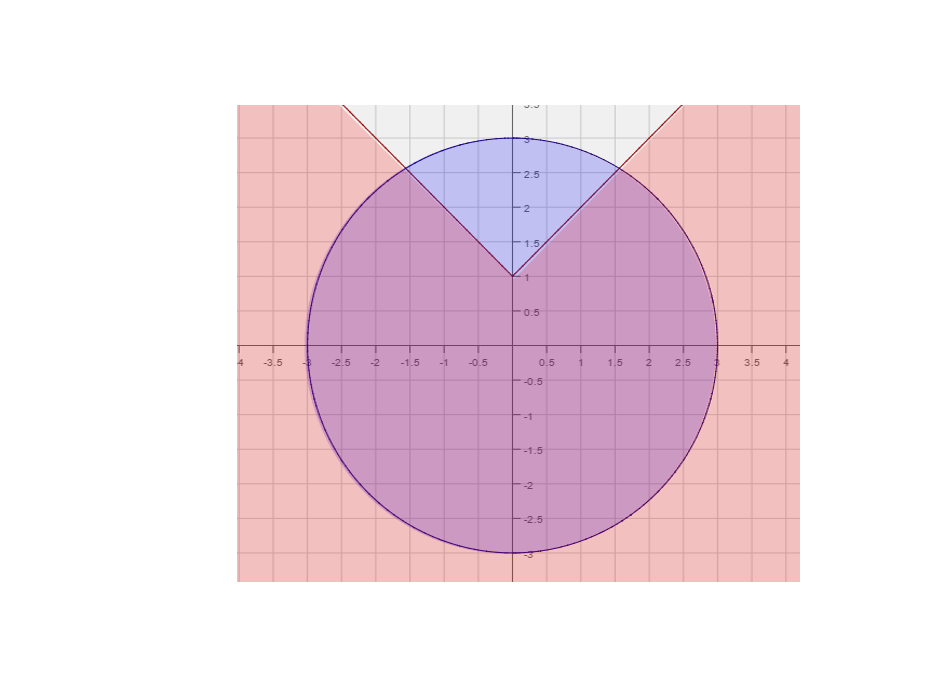# 005 Sample Final A, Question 19

Question Consider the following function,

$f(x)=-\sin \left(3x+{\frac {\pi }{2}}\right)+1$a. What is the amplitude?
b. What is the period?
c. What is the phase shift?
d. What is the vertical shift?
e. Graph one cycle of f(x). Make sure to label five key points.

Foundations:
1) For parts (a) - (d), How do we read the relevant information off of $A\sin(Bx+C)+D?$2) What are the five key points when looking at $\sin(x)?$1) The amplitude is A, the period is ${\frac {2\pi }{B}}$, the horizontal shift is left by C units if C is positive and right by C units if C is negative, the vertical shift is up by D if D is positive and down by D units if D is negative.
2) The five key points are $(0,0),~({\frac {\pi }{2}},1),~(\pi ,0),~({\frac {3\pi }{2}},0),~{\text{and }}(2\pi ,0).$Solution:

Step 1:
Amplitude: -1, period: ${\frac {2\pi }{3}}~$, phase shift: Left by ${\frac {\pi }{2}}~$and vertical shift up by 1.
The five key points for the graph are $(-{\frac {\pi }{6}},0)$The final solution is the portion of the graph that below $y=\vert x\vert +1$and inside $x^{2}+y^{2}=9$